# Continuous in every linear direction not implies continuous

## Statement

### For a function of two variables at a point

It is possible to have a function$f$ of two variables$x,y$ and a point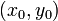$(x_0,y_0)$ in the domain of$f$ such that$f$ is continuous along every linear direction at$(x_0,y_0)$ (i.e., the function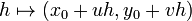$h \mapsto (x_0 + uh, y_0 + vh)$ is continuous at$h = 0$ for every vector$\langle u,v \rangle$) but$f$ is not a continuous function.

### For a function of two variables overall

It is possible to have a function$f$ of two variables$x,y$ such that$f$ is continuous along every linear direction at every point in$\R^2$ but$f$ is not a continuous function everywhere in$\R^2$, i.e., there exists a point in$\R^2$ at which$f$ is not continuous.

### For a function of multiple variables

We can replace functions of two variables by functions of more than two variables.

## Proof

### Example

Consider the function: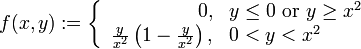$f(x,y) := \left\lbrace \begin{array}{rl} 0, & y \le 0 \mbox{ or } y \ge x^2 \\ \frac{y}{x^2}\left(1 - \frac{y}{x^2}\right), & 0 < y < x^2 \\\end{array}\right.$

Alternatively, we can describe it as: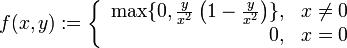$f(x,y) := \left\lbrace \begin{array}{rl} \max \{ 0, \frac{y}{x^2}\left(1 - \frac{y}{x^2}\right) \}, & x \ne 0 \\ 0, & x = 0 \\\end{array}\right.$

We first argue that$f$ is continuous at all points other than$(0,0)$:

• At any point with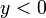$y < 0$, the function is the zero function around the point, hence is continuous.
• At any point with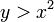$y > x^2$, the function is the zero function around the point, hence is continuous.
• At any point with$0 < y < x^2$, the function has the rational function description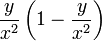$\frac{y}{x^2}\left(1 - \frac{y}{x^2}\right)$ around the point, hence is continuous.
• At any point on the line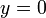$y = 0$ other than the origin, there are two definitions of the function around the point: the definition 0 (from the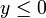$y \le 0$ side), and the definition$\frac{y}{x^2}\left(1 - \frac{y}{x^2}\right)$ (from the$0 < y < x^2$ side). Both definitions evaluate to zero at the point, which is the same as the function value at the point.
• At any point on the curve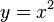$y = x^2$ other than the origin, there are two definitions of the function around the point: the definition 0 (from the$y \ge x^2$ side), and the definition$\frac{y}{x^2}\left(1 - \frac{y}{x^2}\right)$ (from the$0 < y < x^2$ side). Both definitions evaluate to zero at the point, which is the same as the function value at the point.

We now argue that$f$ is continuous in every linear direction at$(0,0)$. It suffices to consider half-line directions because continuity from a linear direction is continuity from both half-line directions.

• For the half-line directions that are below or along the$y = 0$ line, the function is identically zero along the half-line, so the limit at the origin is zero, which equals the zero.
• For the half-line direction the positive$y$-axis, the function is identically zero along the half-line, so the limit at the origin is zero, which equals the zero.
• For the other half-line directions that are above$y = 0$, we note that, sufficiently close to the origin, this half-line is completely above the$y = x^2$ curve (explicitly, if the slope of the line is$m$, then the half-line is above$y = x^2$ for$|x| < |m|$). Thus, sufficiently close to the origin,$f$ looks like the zero function on this half-line. Thus, the limit at the origin is zero, which equals the value.

We finally demonstrate that$f$ is not continuous at$(0,0)$ by finding a curve approaching the origin along which the limit at the origin is not zero. Consider the curve:$y = \frac{1}{2}x^2$

Consider the limit:$\lim_{x \to 0^+} f(x,y) = \lim_{x \to 0^+} f(x,x^2/2) = \lim_{x \to 0^+} (1/2)(1 - 1/2) = \lim_{x \to 0^+} 1/4 = 1/4 \ne 0$

### Intuitive explanation of example

Intuitively, this example function is zero on a very large subset of the domain, and the set of points where it is nonzero is a narrow squished subset of the plane that, near the origin, is too small to be detected by straight lines.

### Generalization to functions of more than two variables

We can use the same example as for a function of two variables, with the above functional form in terms of two of the input variables, and with the function independent of the remaining variables.